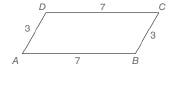Chapter 4.2, Problem 1E### Elementary Geometry for College St...

6th Edition
Daniel C. Alexander + 1 other
ISBN: 9781285195698

#### Solutions

Chapter
Section### Elementary Geometry for College St...

6th Edition
Daniel C. Alexander + 1 other
ISBN: 9781285195698
Textbook Problem
1 views

# Note: Exercises preceded by an asterisk are of a more challenging nature.a) As shown, must quadrilateral ABCD be a parallelogram? b ) Given the lengths of the sides as shown, is the measure of ∠ A unique?To determine

a)

To check:

Whether the given quadrilateral is a parallelogram.

Explanation

Given,

AB=7 units

BC=3 units

CD=7 units

DA=3 units and

Draw the diagonal AC¯ as exactly one line passes through two points.

Now, we have two triangles ABC and CDA, such that

AC<

To determine

b)

Whether the measure of A is unique for the given lengths of the sides.

### Still sussing out bartleby?

Check out a sample textbook solution.

See a sample solution

#### The Solution to Your Study Problems

Bartleby provides explanations to thousands of textbook problems written by our experts, many with advanced degrees!

Get Started

#### Solve

Mathematical Applications for the Management, Life, and Social Sciences

#### Convert the expressions in Exercises 6584 to power form. 3x453x+43xx

Finite Mathematics and Applied Calculus (MindTap Course List)

#### Let h be the function defined by h(x) = x3 x2 + x + 1. Find h(5), h(0), h(a), and h(a).

Applied Calculus for the Managerial, Life, and Social Sciences: A Brief Approach